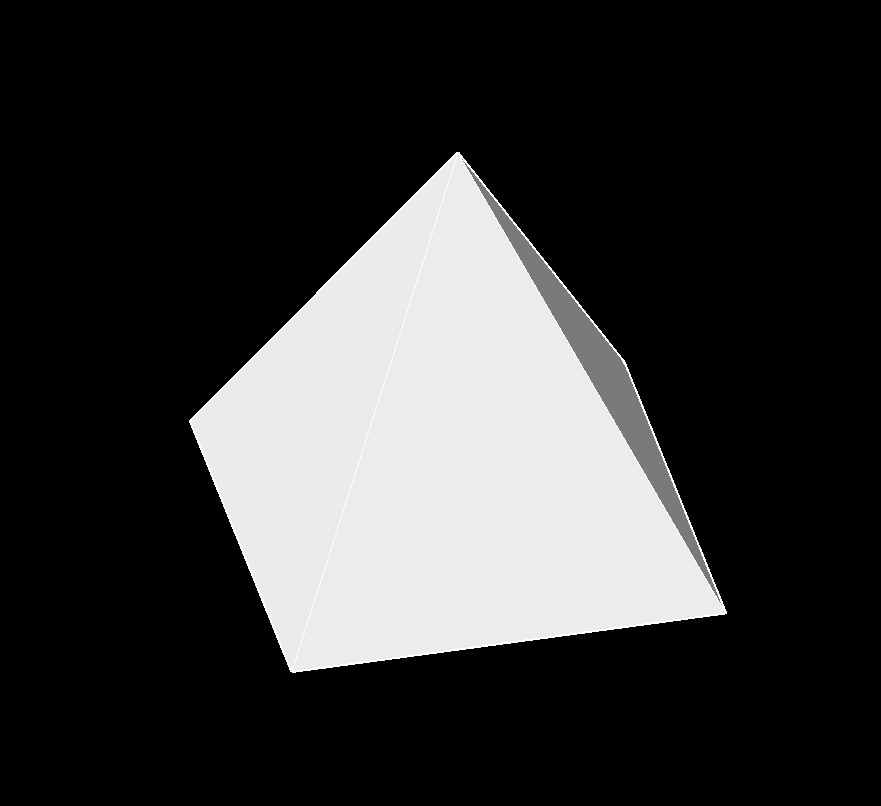# Right Square Pyramid

Written by Jerry Ratzlaff on . Posted in Solid Geometry

•Right square pyramid (a three-dimensional figure) has a square base and the apex alligned above the center of the base.
• 1 base
• 8 edges
• 4 faces
• 5 vertexs

## formulas that use Base Area of a Right Square Pyramid

 $$\large{ A_b = a^2 }$$

### Where:

$$\large{ A_b }$$ = base area

$$\large{ a }$$ = edge

## formulas that use Edge of a Right Square Pyramid

 $$\large{ a = \sqrt{ 3\; \frac{V}{h} } }$$

### Where:

$$\large{ a }$$ = edge

$$\large{ h }$$ = height

$$\large{ V }$$ = volume

## formulas that use Height of a Right Square Pyramid

 $$\large{ h = \frac{1}{2} \; \sqrt{ 16\; \left( \frac{A_f}{a} \right)^2 - a^2 } }$$

### Where:

$$\large{ h }$$ = height

$$\large{ a }$$ = edge

$$\large{ V }$$ = volume

## formulas that use Face Area of a Right Square Pyramid

 $$\large{ A_f = \frac{a}{2} \; \sqrt{\frac{a^2}{4} +h^2 } }$$

### Where:

$$\large{ A_f}$$ = face area (side)

$$\large{ a }$$ = edge

$$\large{ h }$$ = height

## formulas that use Lateral Surface Area of a Right Square Pyramid

 $$\large{ A_l = a \sqrt {a^2 + 4\;h^2 } }$$

### Where:

$$\large{ A_l }$$ = lateral surface area (sides)

$$\large{ a }$$ = edge

$$\large{ h }$$ = height

## formulas that use Surface Area of a Right Square Pyramid

 $$\large{ A_s= a^2+2\;a\; \sqrt {\frac {a^2} {4} +h^2 } }$$

### Where:

$$\large{ A_s }$$ = surface area (bottom, sides)

$$\large{ a }$$ = edge

$$\large{ h }$$ = edge

## formulas that use Volume of a Right Square Pyramid

 $$\large{ V= a^2\; \frac{h}{3} }$$

### Where:

$$\large{ V }$$ = volume

$$\large{ a }$$ = edge

$$\large{ h }$$ = height